# RD Sharma Solutions for Class 11 Chapter 33 - Probability Exercise 33.3

This exercise deals with the axiomatic approach to probability that is deduced from the mathematical concepts. It is based upon certain axioms. The main aim of experts is to provide solutions and thereby help students, irrespective of their intelligence quotient. The solutions are explained and solved in simple language to help them perform well in the board exams. Students are advised to solve the exercise wise problems on a daily basis to understand the concepts in a better way. Students can download RD Sharma Class 11 Maths Solutions from the links given below and can use it for future reference as well.

## Download the pdf of RD Sharma Solutions for Class 11 Maths Exercise 33.3 Chapter 33 – Probability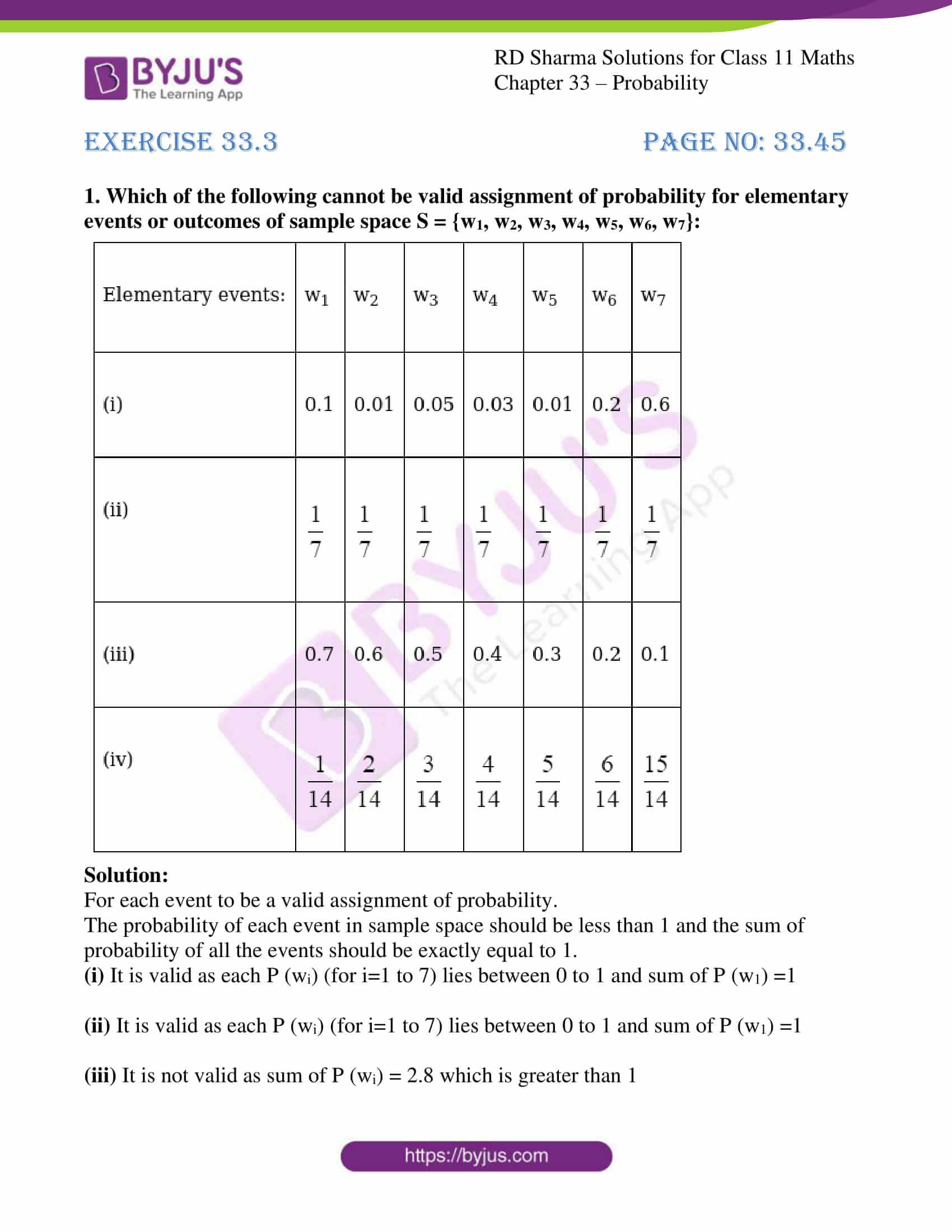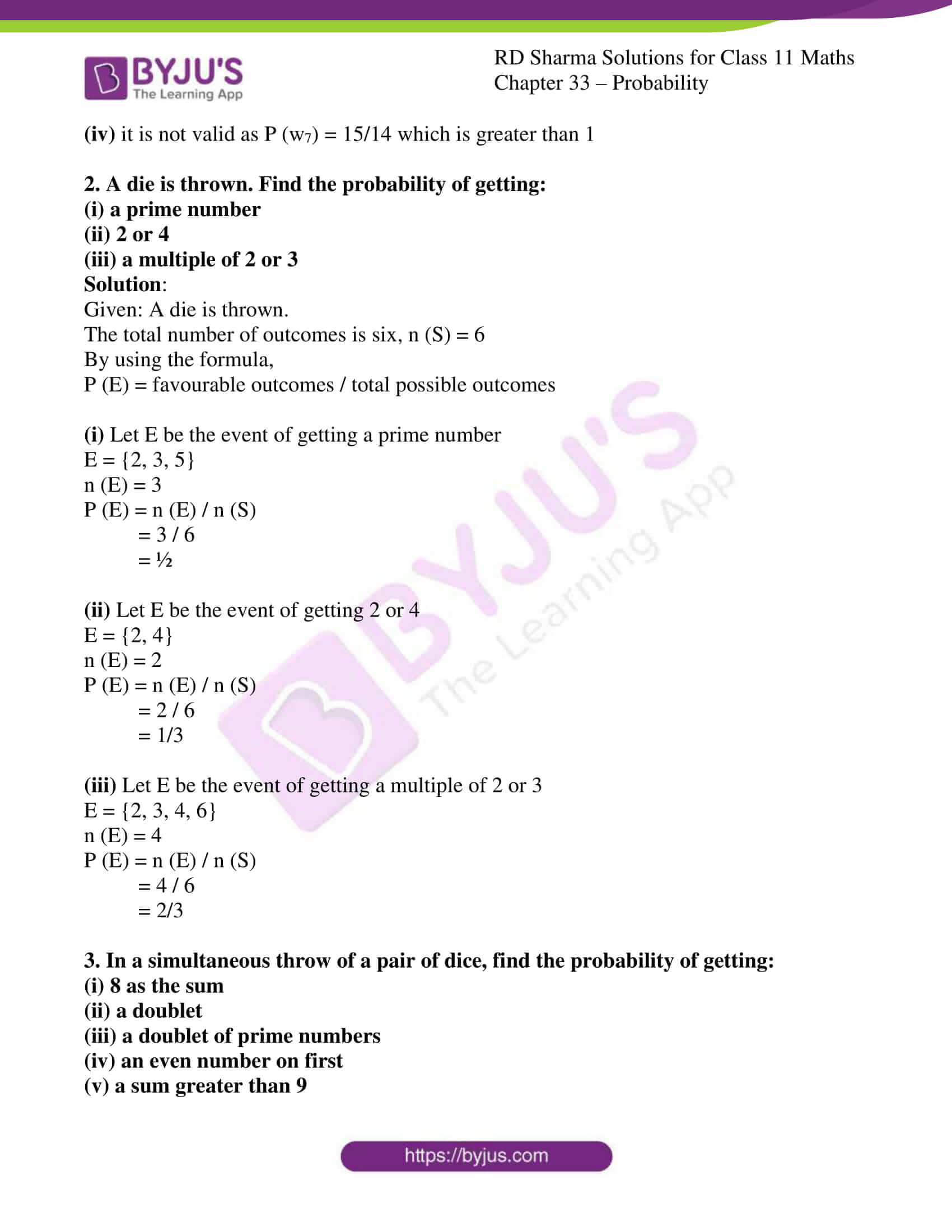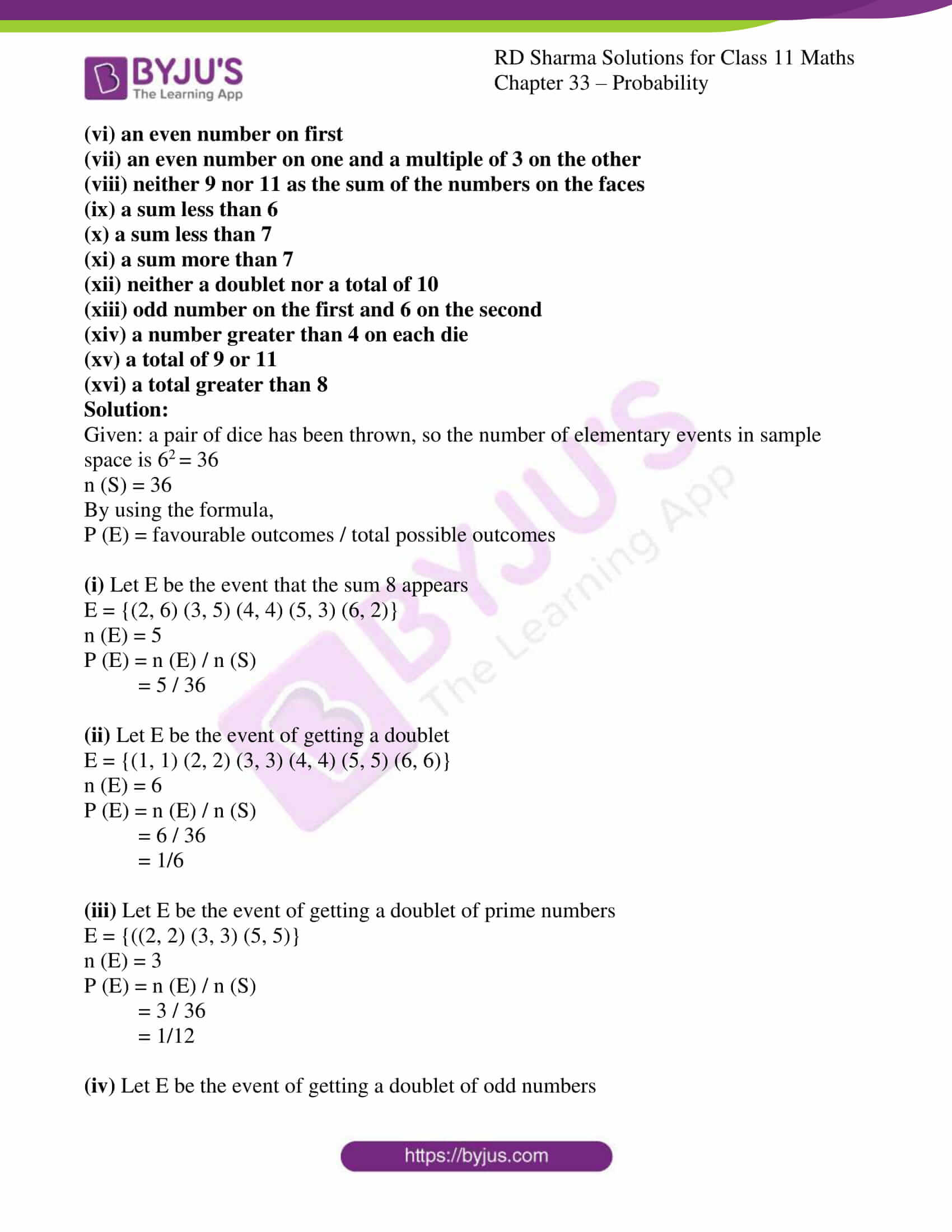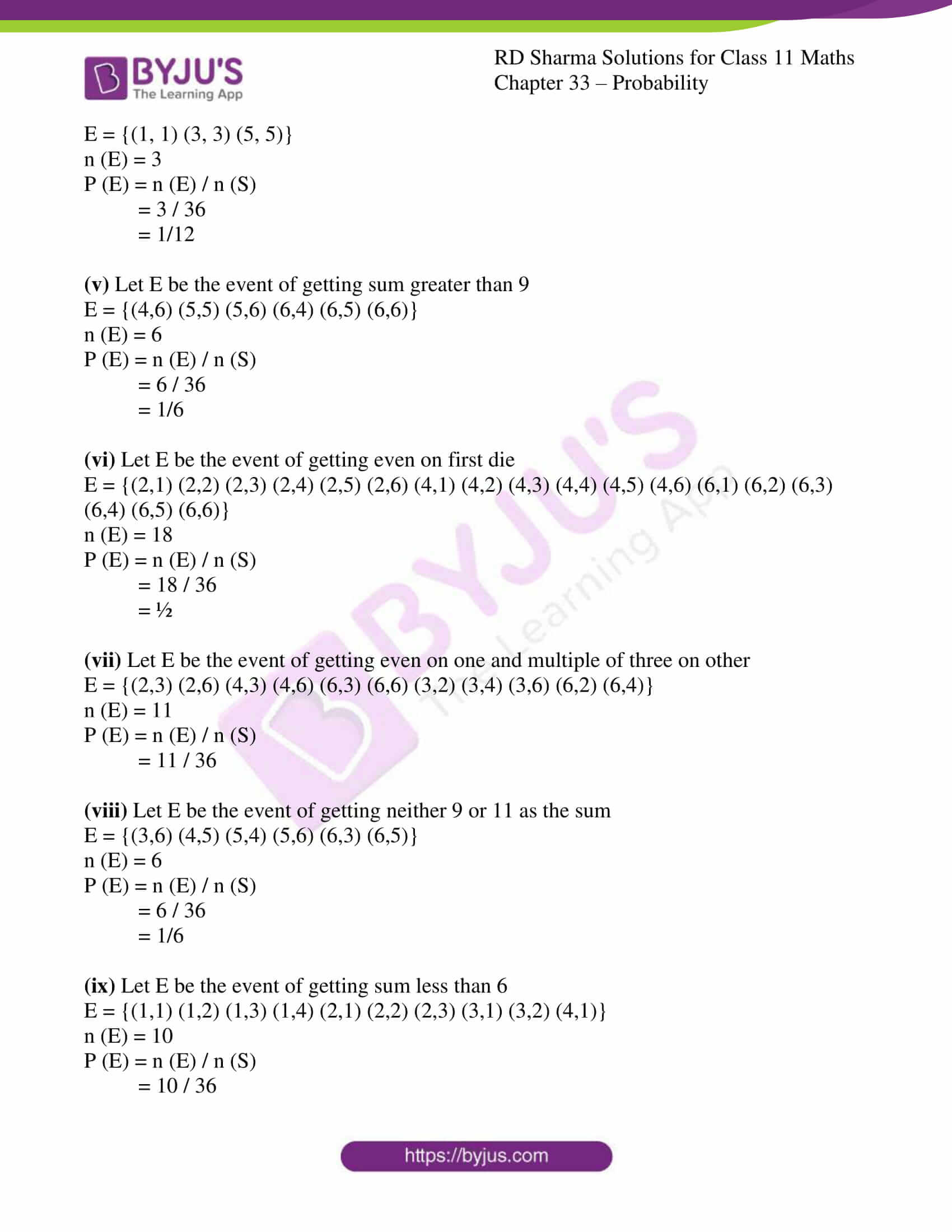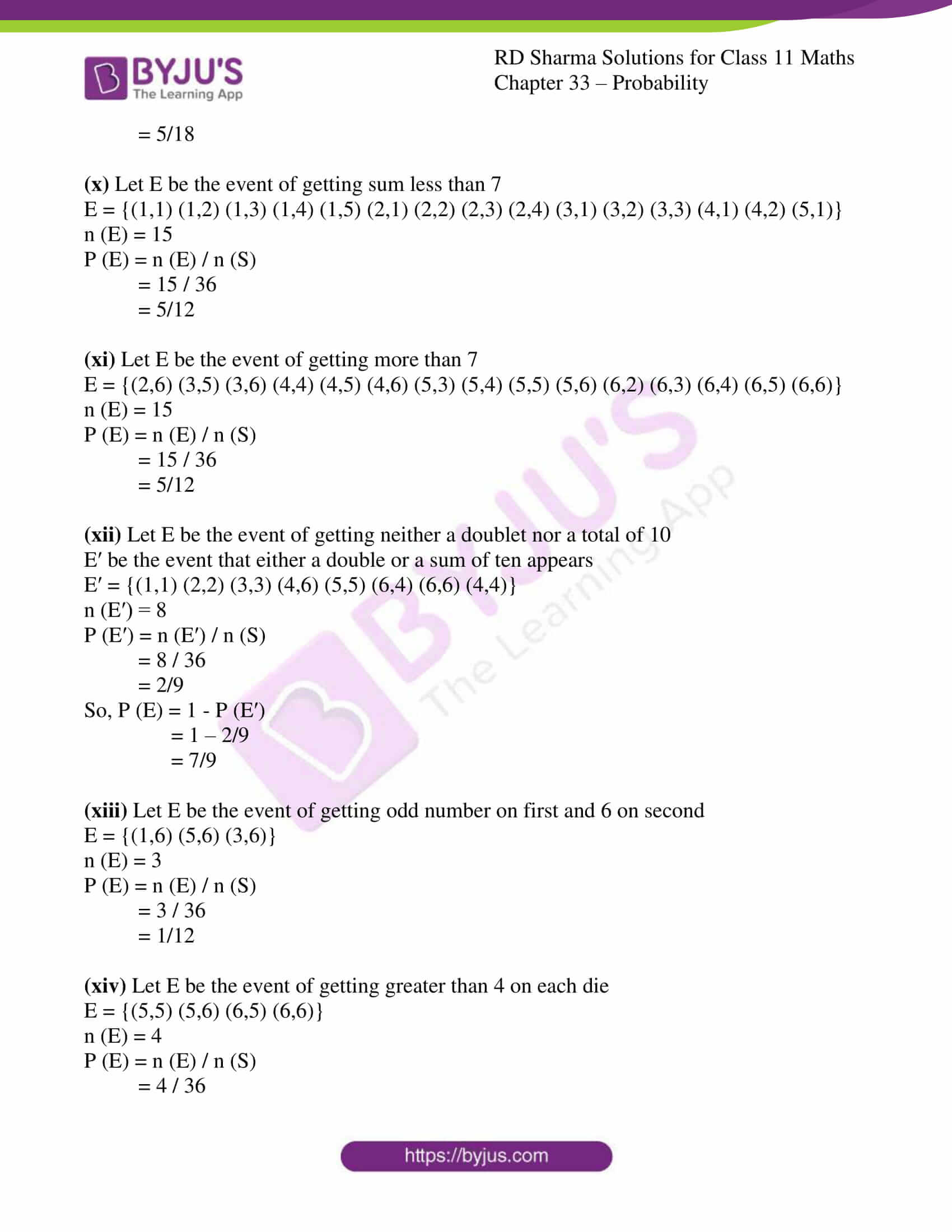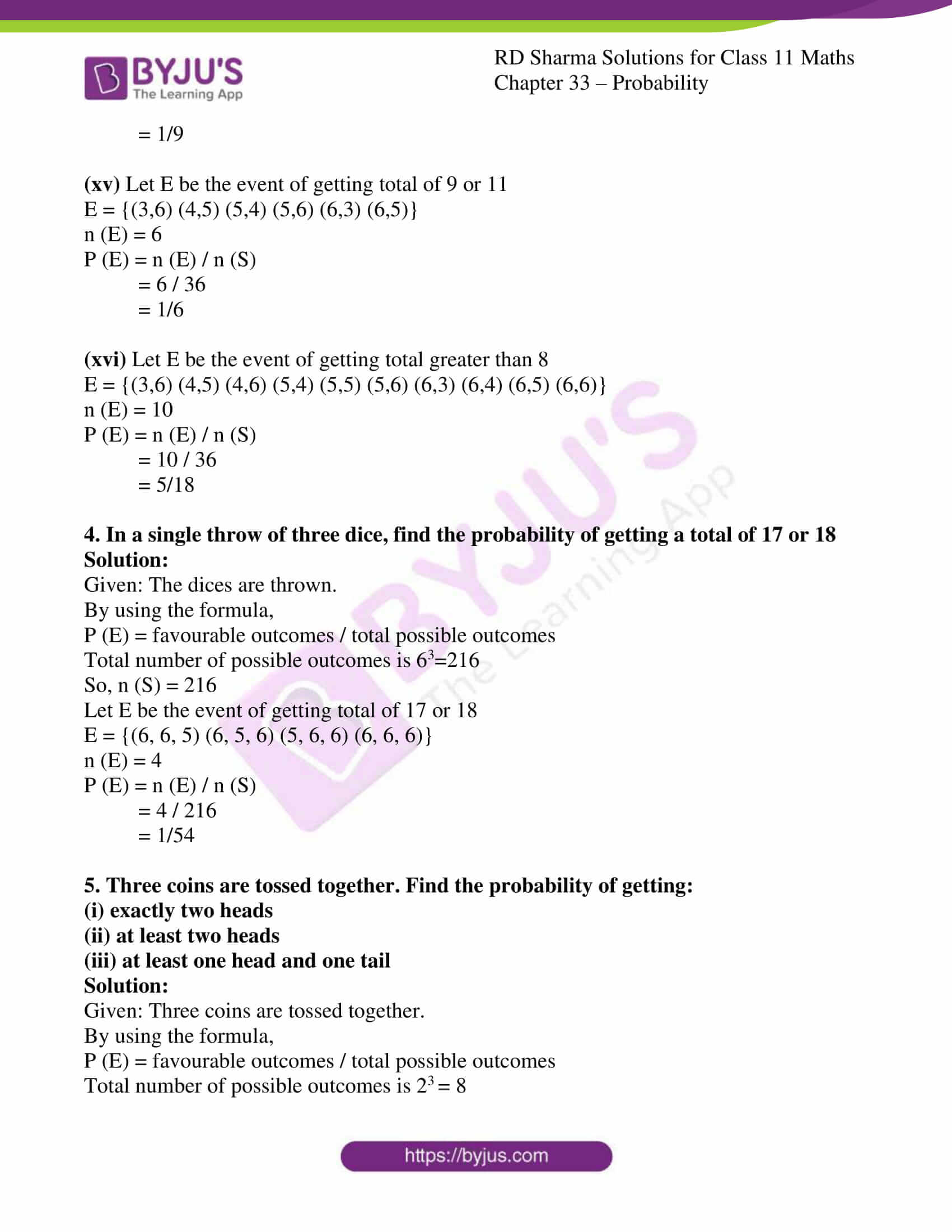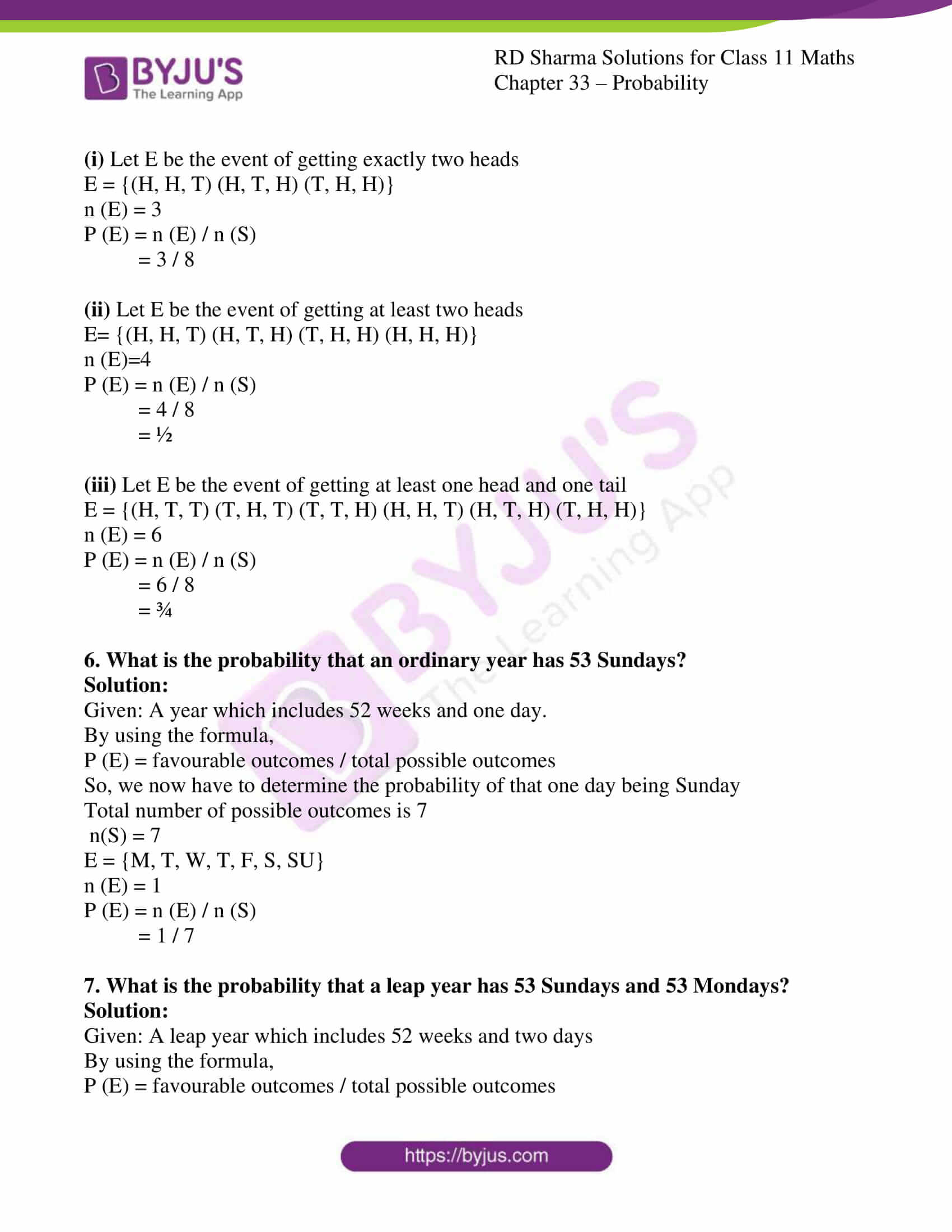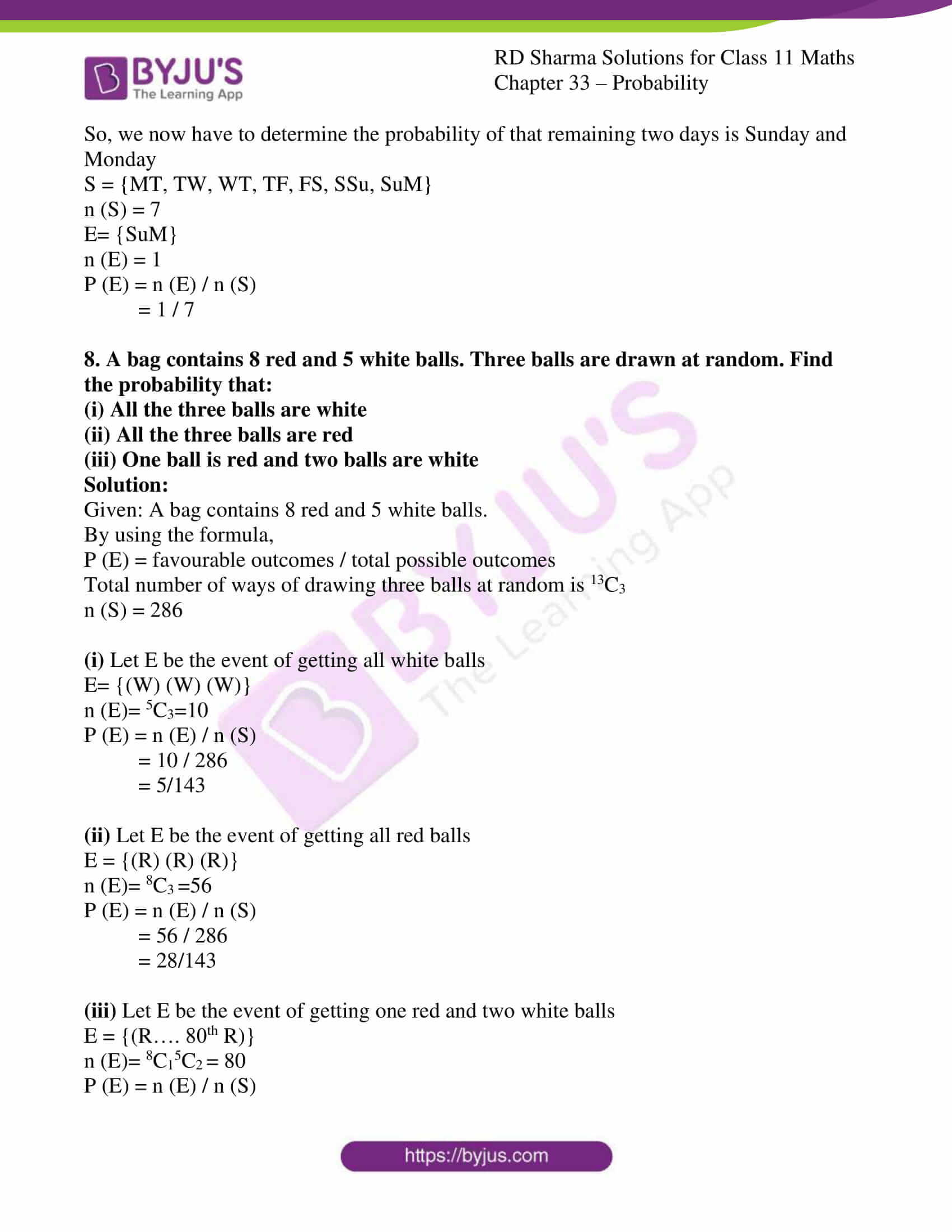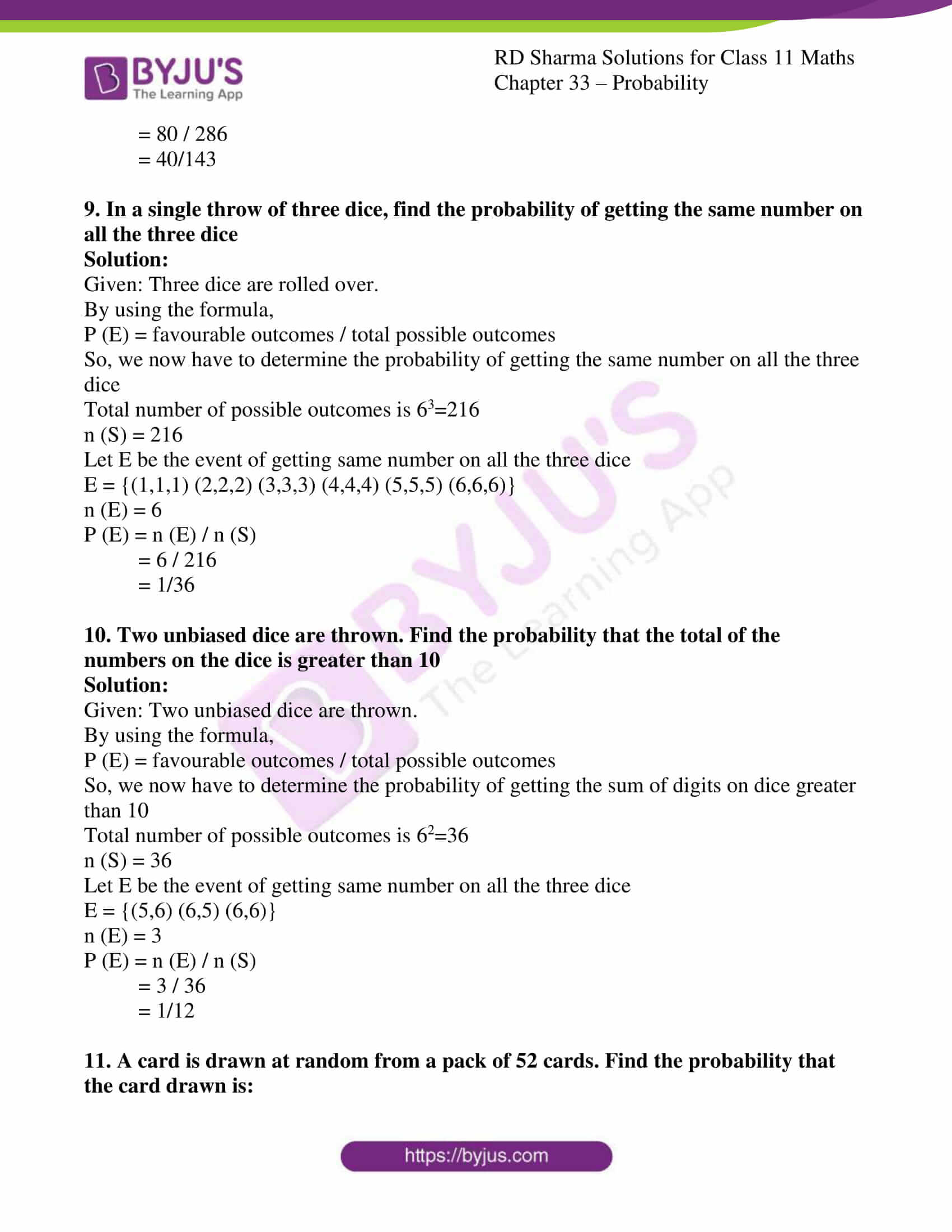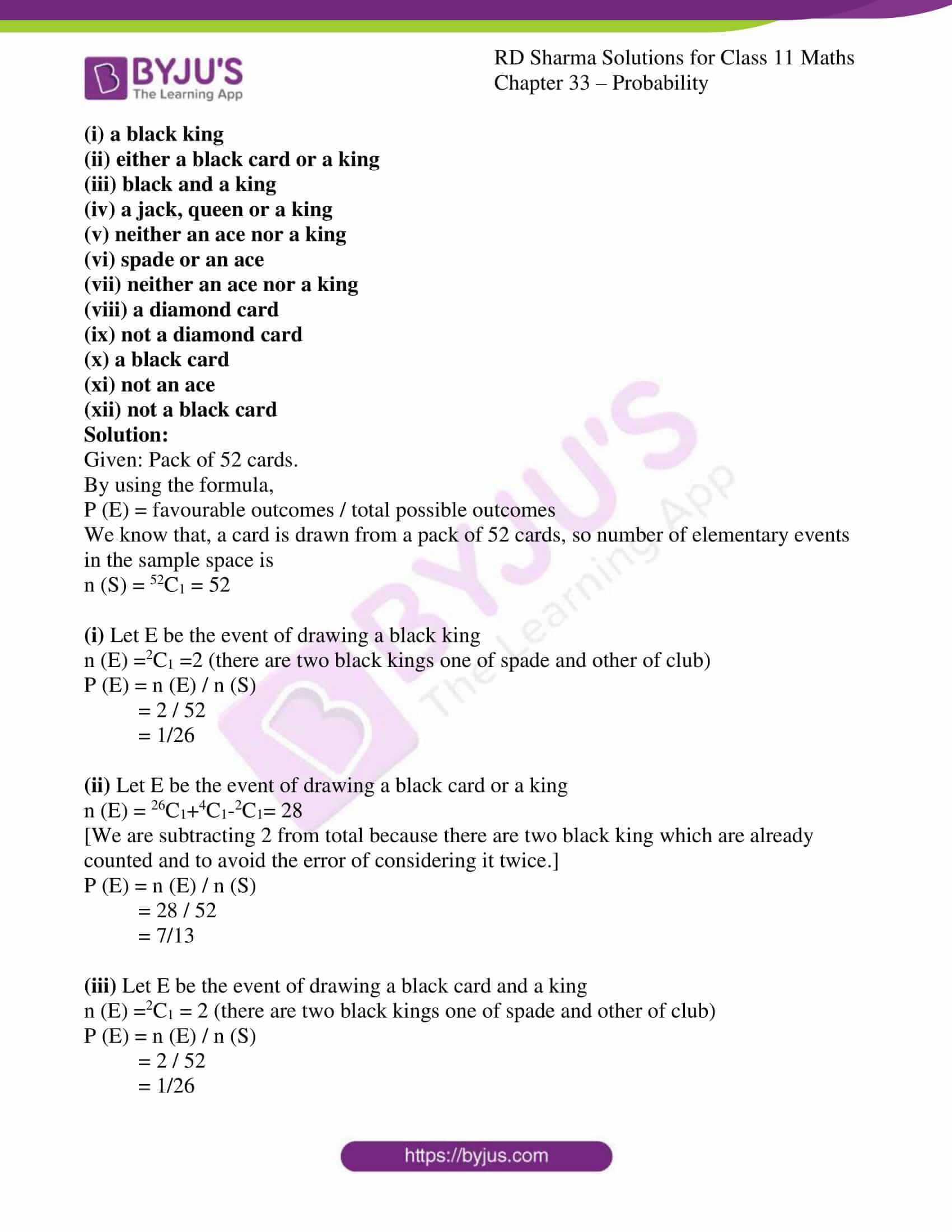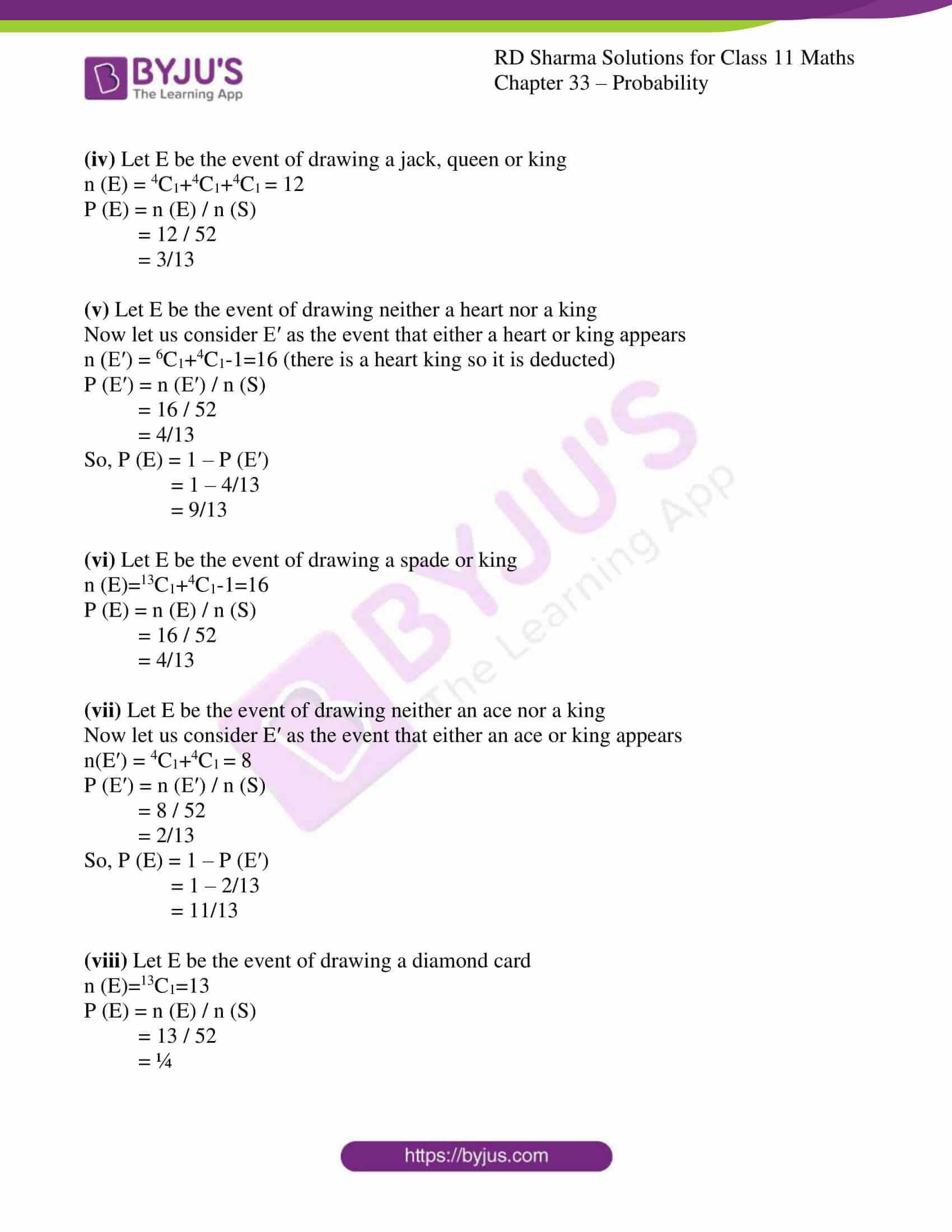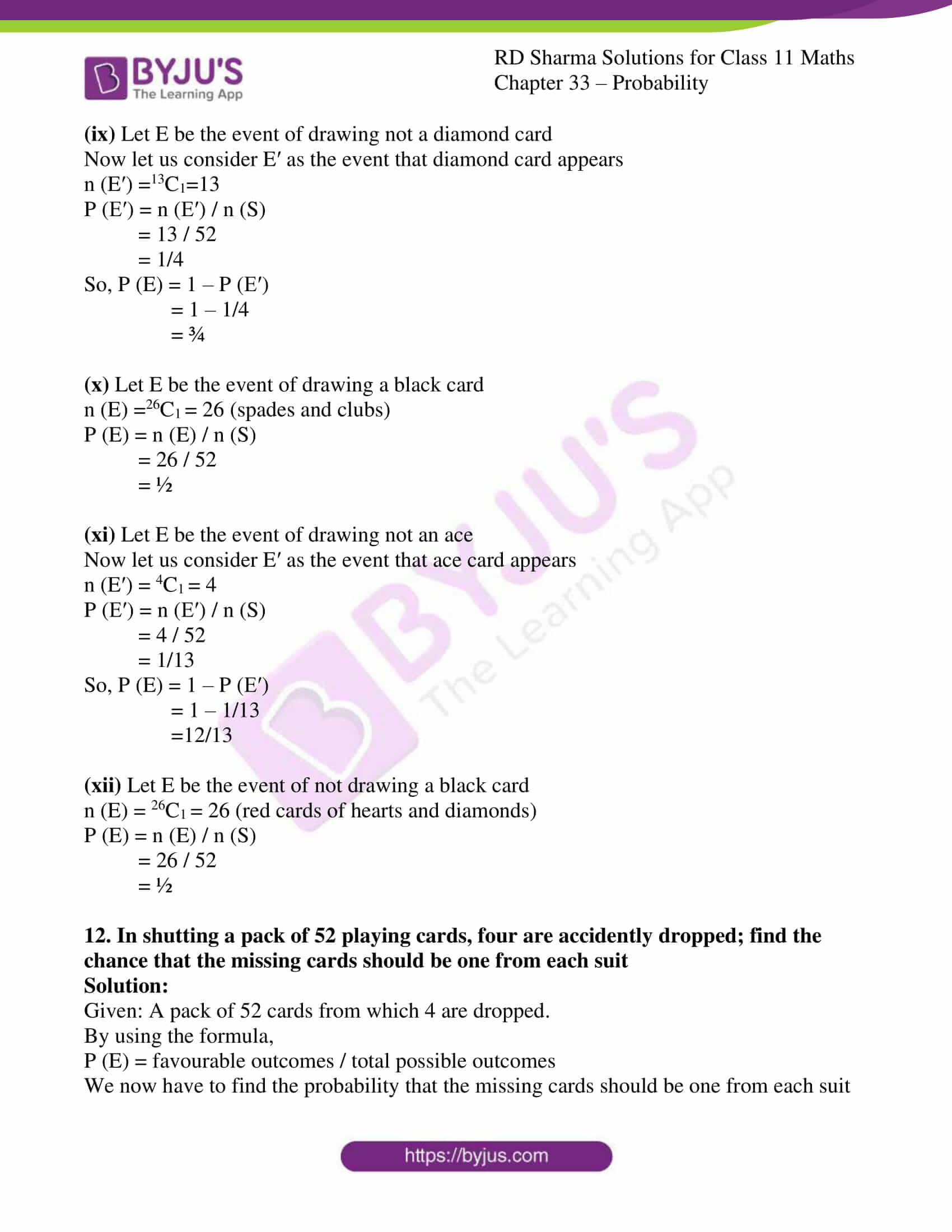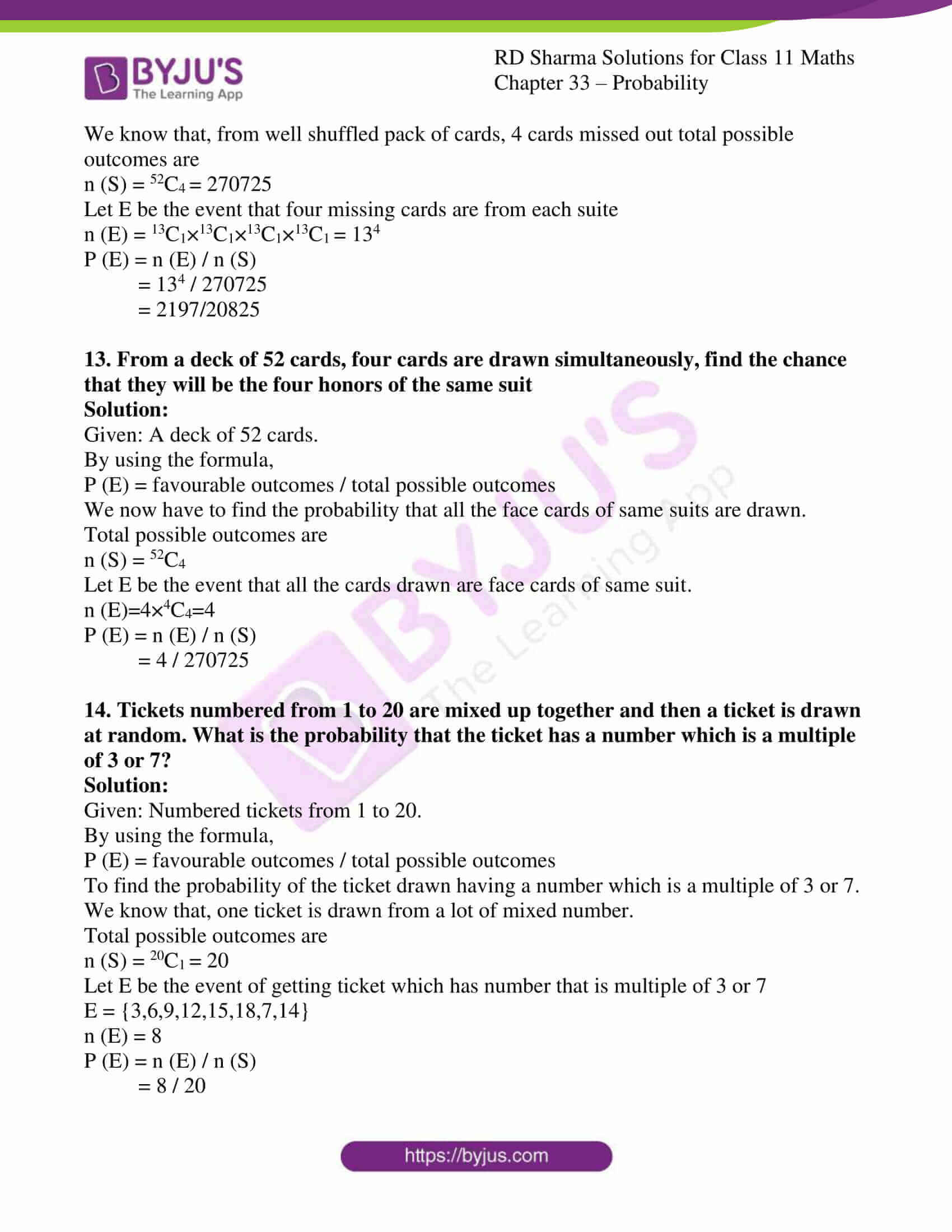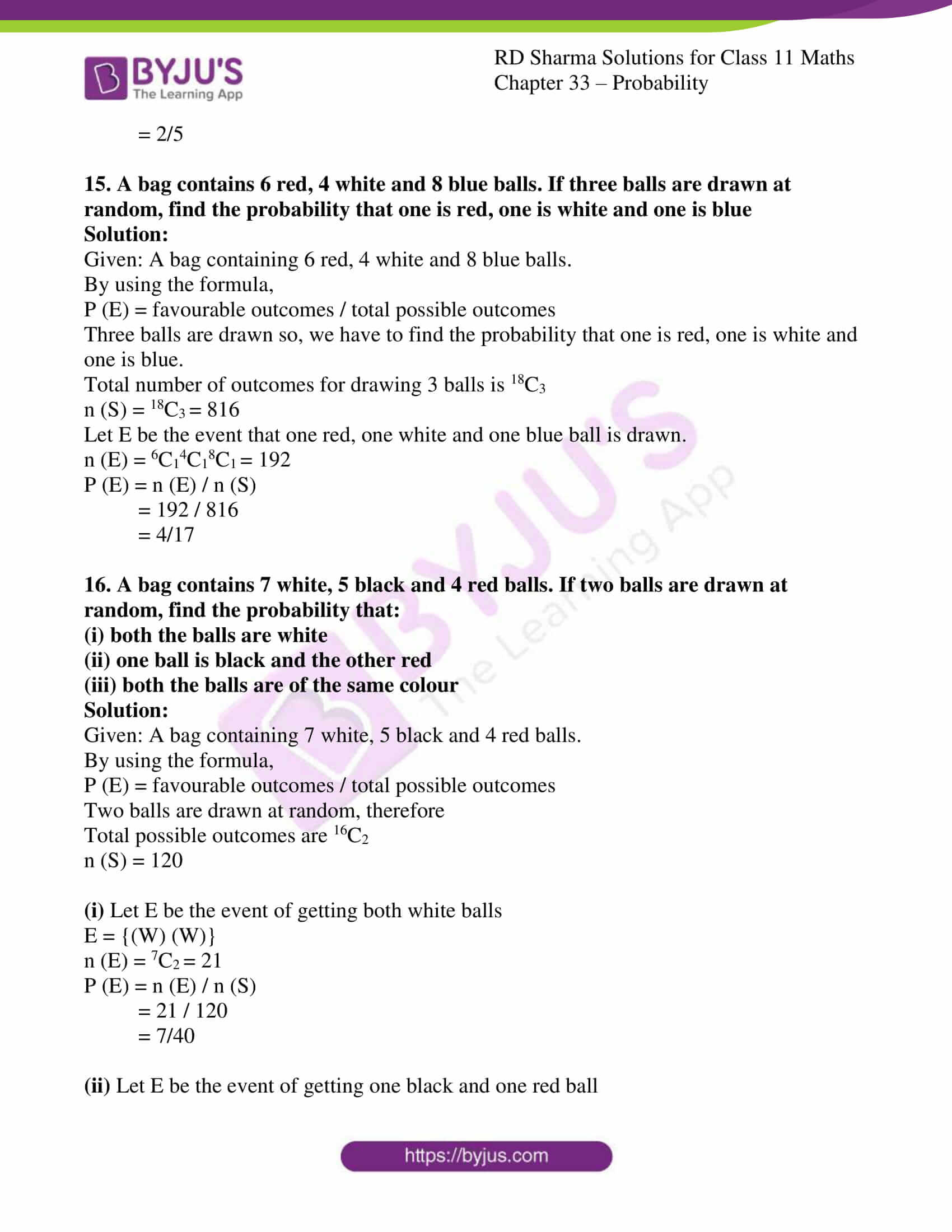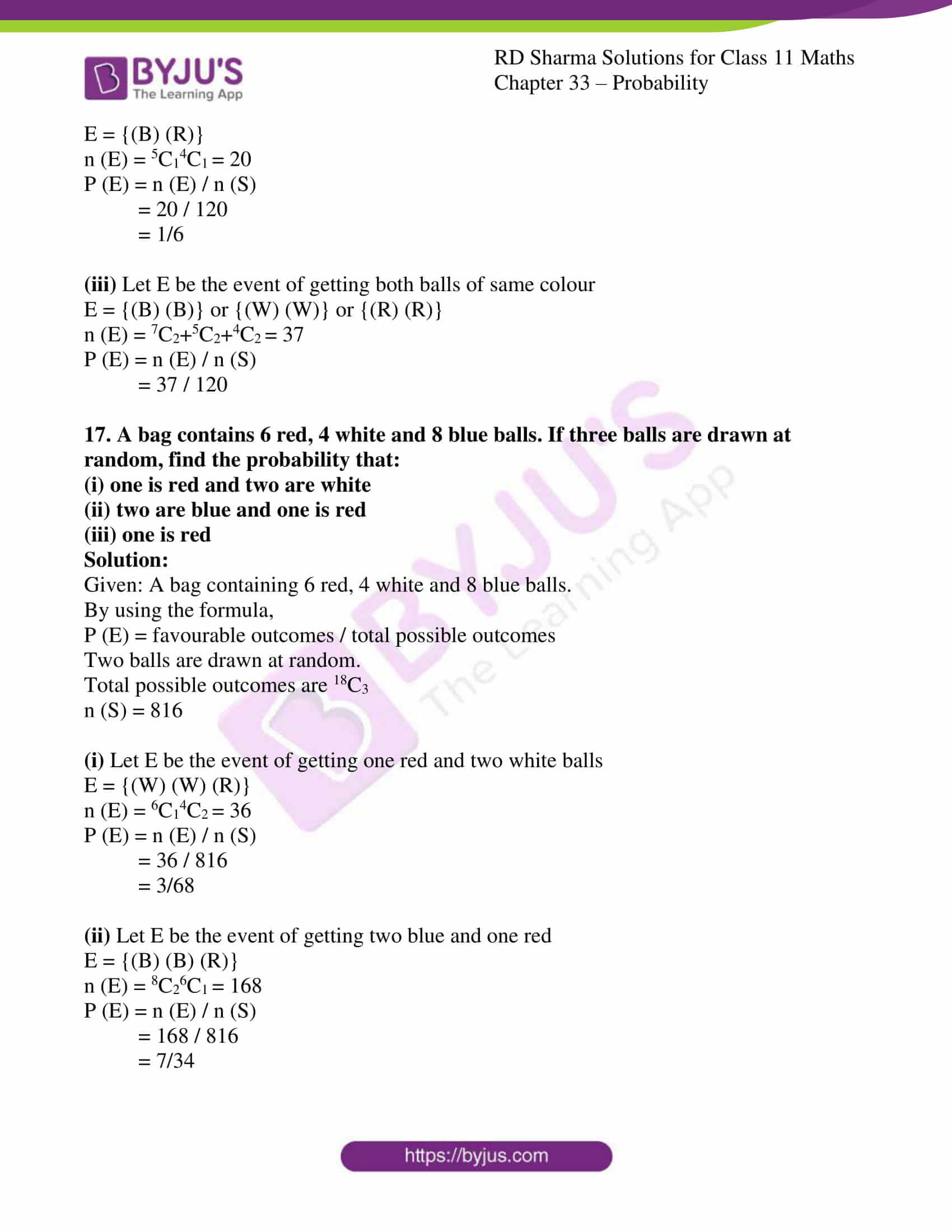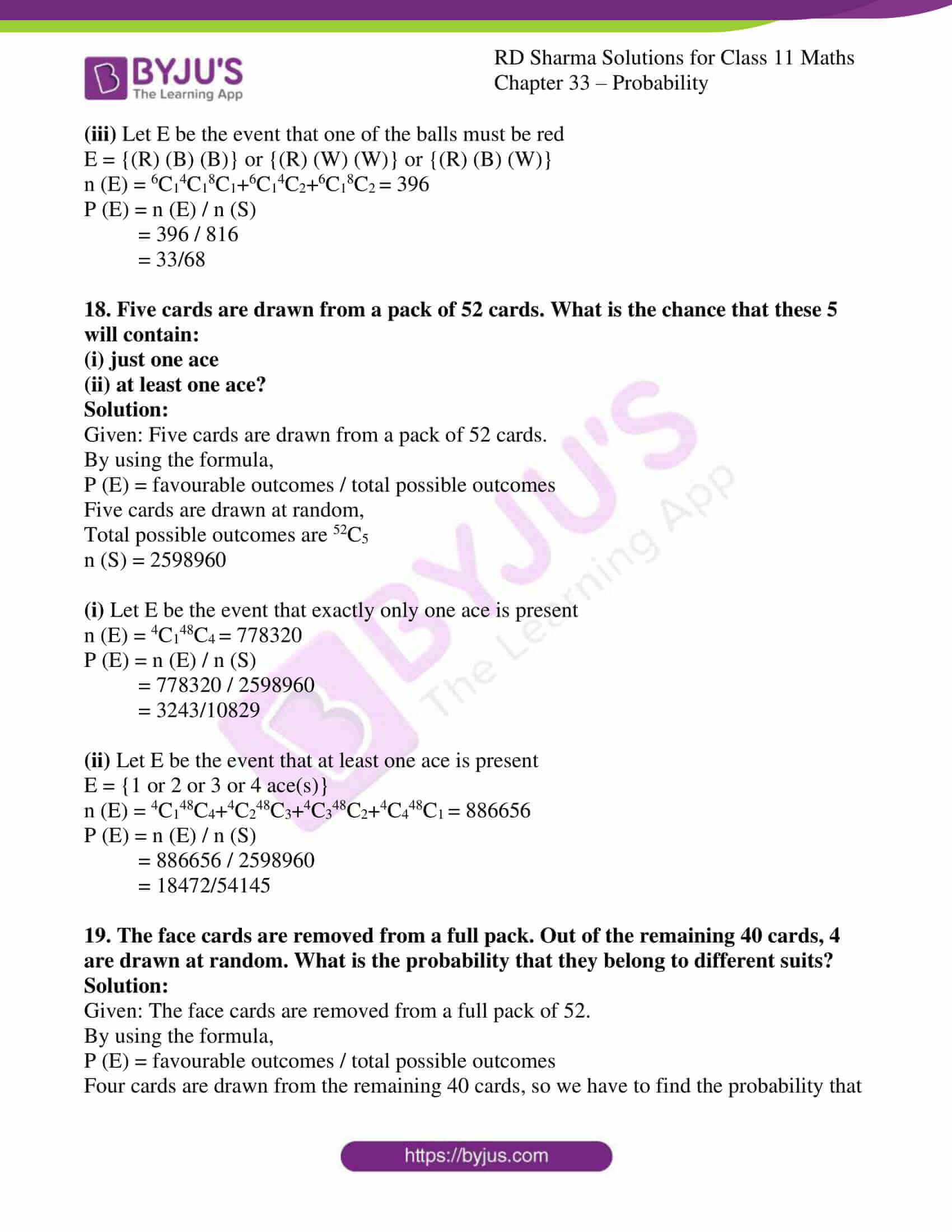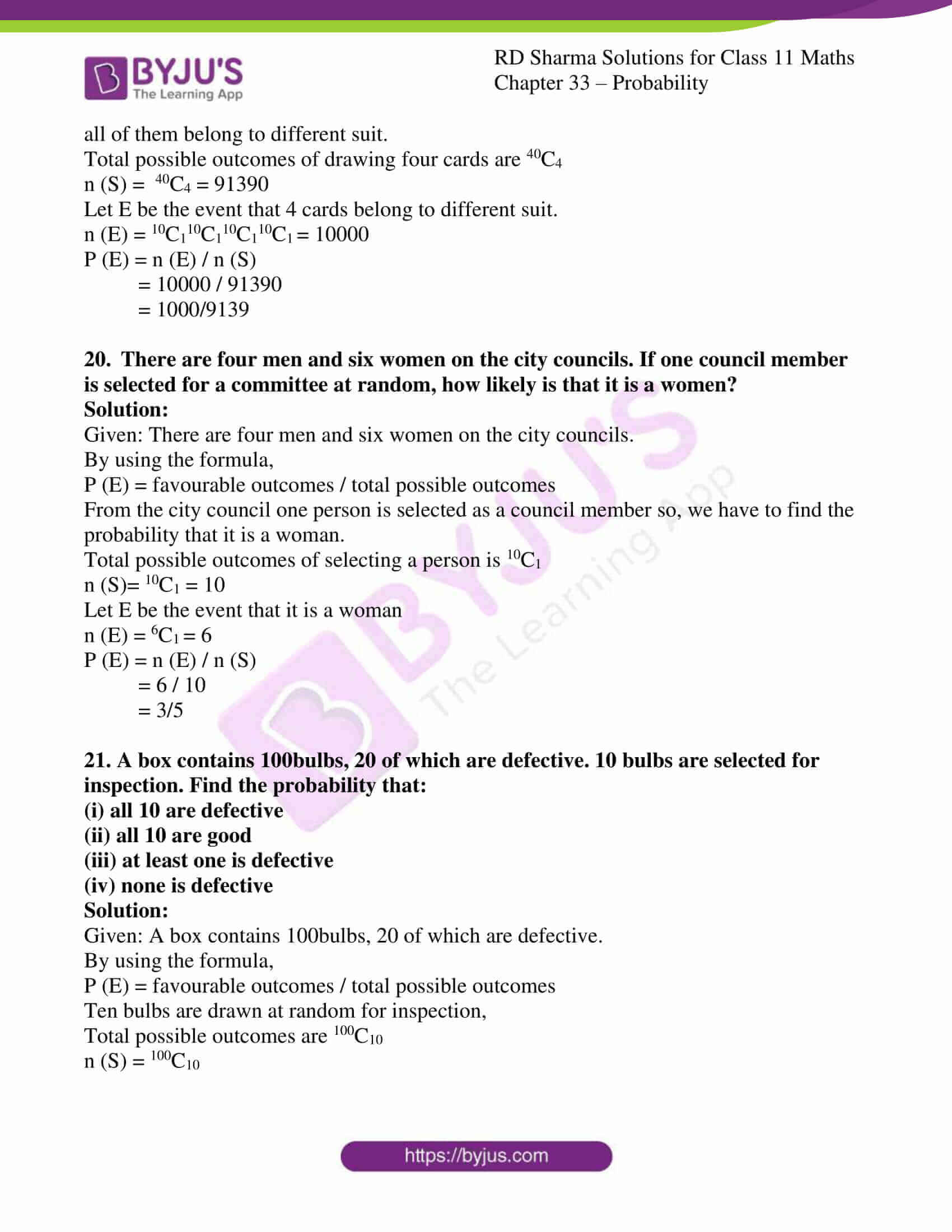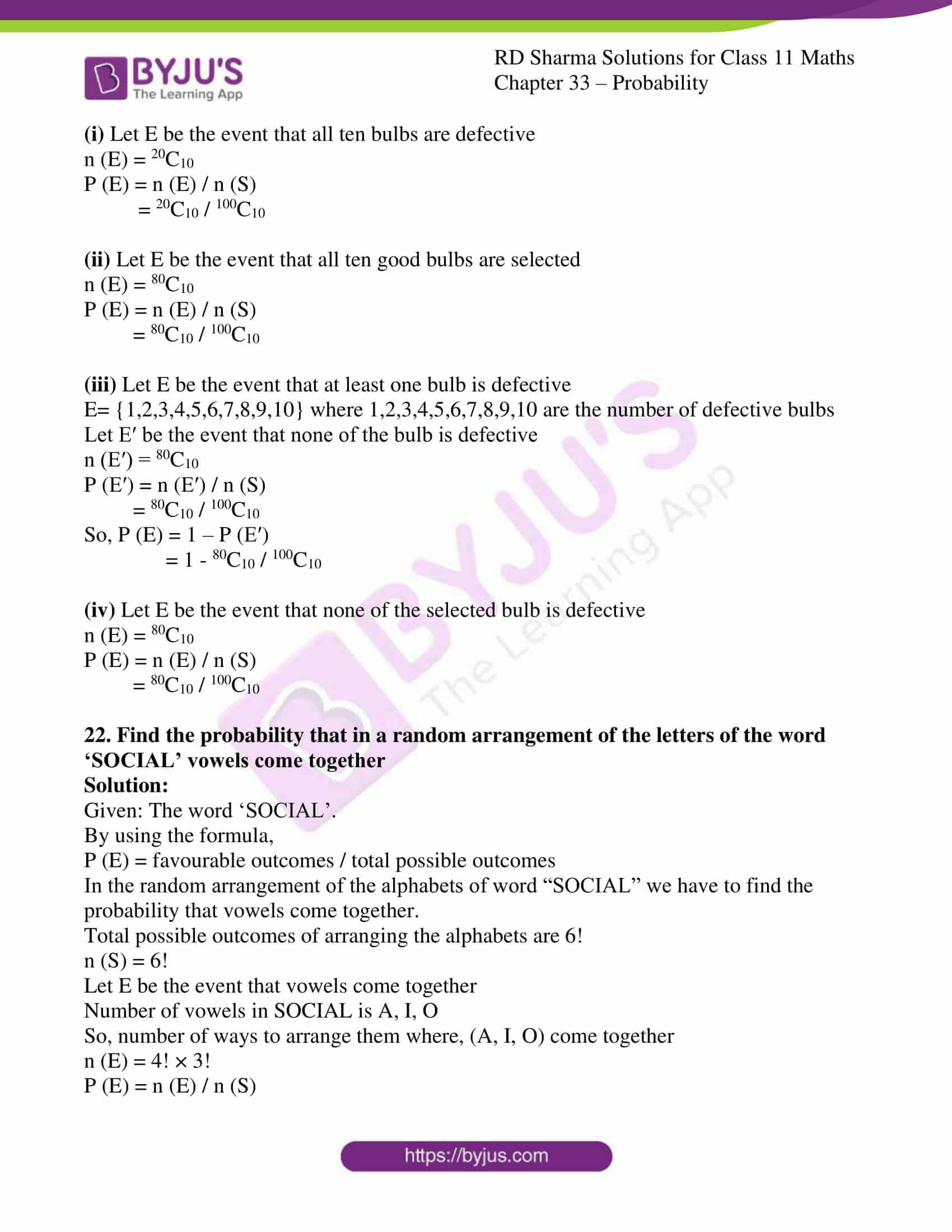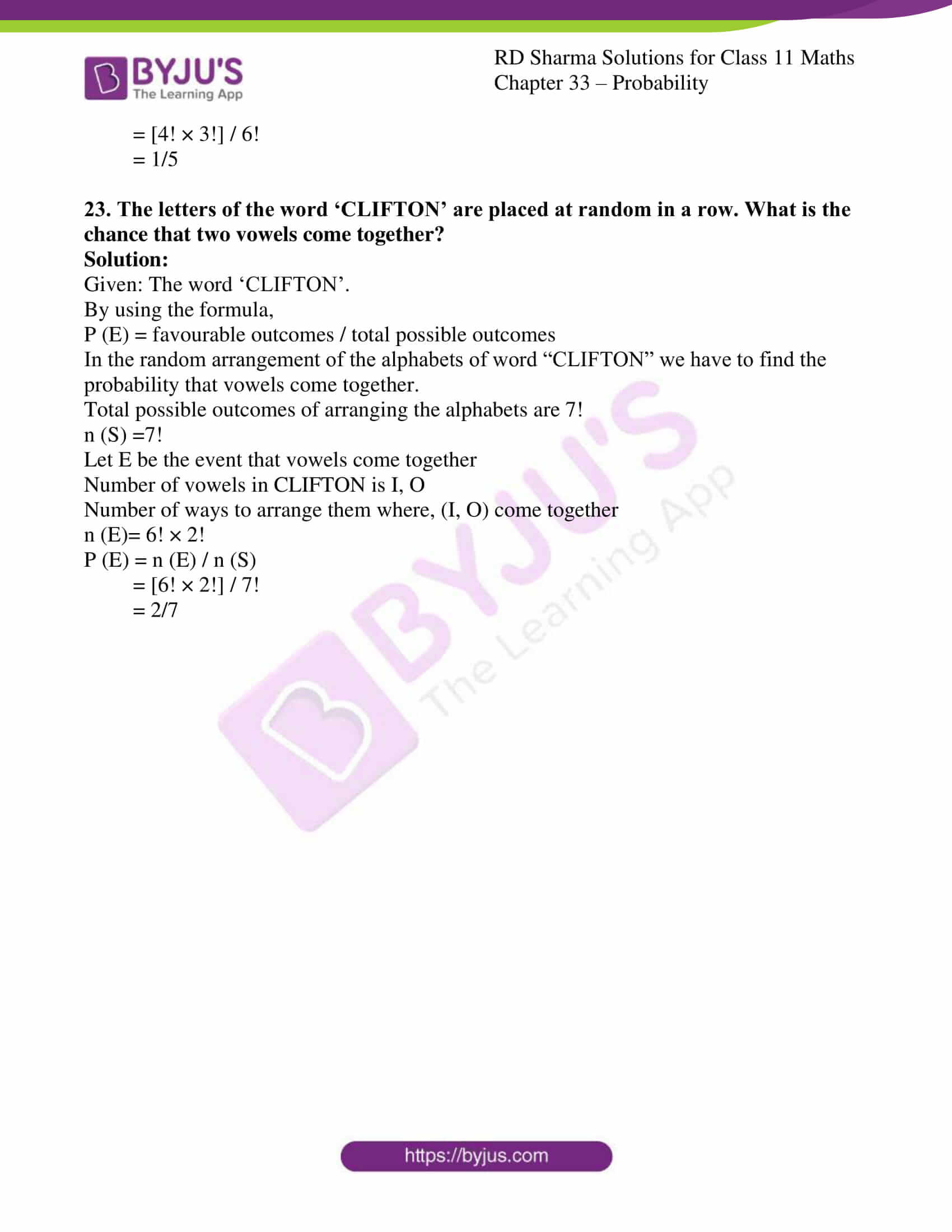### Access answers to RD Sharma Solutions for Class 11 Maths Exercise 33.3 Chapter 33 – Probability

#### EXERCISE 33.3 PAGE NO: 33.45

1. Which of the following cannot be valid assignment of probability for elementary events or outcomes of sample space S = {w1, w2, w3, w4, w5, w6, w7}: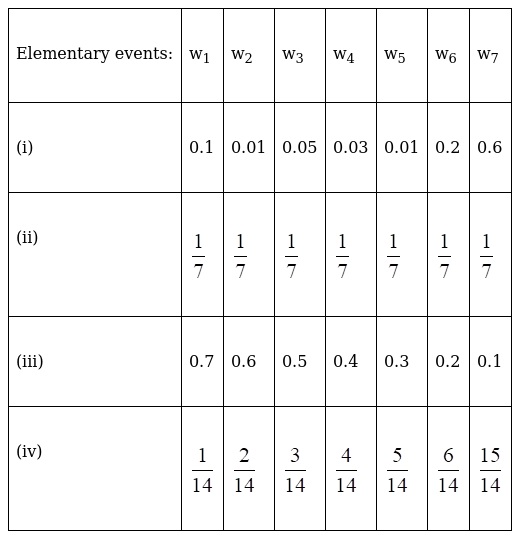Solution:

For each event to be a valid assignment of probability.

The probability of each event in sample space should be less than 1 and the sum of probability of all the events should be exactly equal to 1.

(i) It is valid as each P (wi) (for i=1 to 7) lies between 0 to 1 and sum of P (w1) =1

(ii) It is valid as each P (wi) (for i=1 to 7) lies between 0 to 1 and sum of P (w1) =1

(iii) It is not valid as sum of P (wi) = 2.8 which is greater than 1

(iv) it is not valid as P (w7) = 15/14 which is greater than 1

2. A die is thrown. Find the probability of getting:
(i) a prime number
(ii) 2 or 4
(iii) a multiple of 2 or 3

Solution:

Given: A die is thrown.

The total number of outcomes is six, n (S) = 6

By using the formula,

P (E) = favourable outcomes / total possible outcomes

(i) Let E be the event of getting a prime number

E = {2, 3, 5}

n (E) = 3

P (E) = n (E) / n (S)

= 3 / 6

= ½

(ii) Let E be the event of getting 2 or 4

E = {2, 4}

n (E) = 2

P (E) = n (E) / n (S)

= 2 / 6

= 1/3

(iii) Let E be the event of getting a multiple of 2 or 3

E = {2, 3, 4, 6}

n (E) = 4

P (E) = n (E) / n (S)

= 4 / 6

= 2/3

3. In a simultaneous throw of a pair of dice, find the probability of getting:
(i) 8 as the sum
(ii) a doublet
(iii) a doublet of prime numbers
(iv) an even number on first
(v) a sum greater than 9
(vi) an even number on first
(vii) an even number on one and a multiple of 3 on the other
(viii) neither 9 nor 11 as the sum of the numbers on the faces
(ix) a sum less than 6
(x) a sum less than 7
(xi) a sum more than 7
(xii) neither a doublet nor a total of 10
(xiii) odd number on the first and 6 on the second
(xiv) a number greater than 4 on each die
(xv) a total of 9 or 11
(xvi) a total greater than 8

Solution:

Given: a pair of dice has been thrown, so the number of elementary events in sample space is 62 = 36

n (S) = 36

By using the formula,

P (E) = favourable outcomes / total possible outcomes

(i) Let E be the event that the sum 8 appears

E = {(2, 6) (3, 5) (4, 4) (5, 3) (6, 2)}

n (E) = 5

P (E) = n (E) / n (S)

= 5 / 36

(ii) Let E be the event of getting a doublet

E = {(1, 1) (2, 2) (3, 3) (4, 4) (5, 5) (6, 6)}

n (E) = 6

P (E) = n (E) / n (S)

= 6 / 36

= 1/6

(iii) Let E be the event of getting a doublet of prime numbers

E = {((2, 2) (3, 3) (5, 5)}

n (E) = 3

P (E) = n (E) / n (S)

= 3 / 36

= 1/12

(iv) Let E be the event of getting a doublet of odd numbers

E = {(1, 1) (3, 3) (5, 5)}

n (E) = 3

P (E) = n (E) / n (S)

= 3 / 36

= 1/12

(v) Let E be the event of getting sum greater than 9

E = {(4,6) (5,5) (5,6) (6,4) (6,5) (6,6)}

n (E) = 6

P (E) = n (E) / n (S)

= 6 / 36

= 1/6

(vi) Let E be the event of getting even on first die

E = {(2,1) (2,2) (2,3) (2,4) (2,5) (2,6) (4,1) (4,2) (4,3) (4,4) (4,5) (4,6) (6,1) (6,2) (6,3) (6,4) (6,5) (6,6)}

n (E) = 18

P (E) = n (E) / n (S)

= 18 / 36

= ½

(vii) Let E be the event of getting even on one and multiple of three on other

E = {(2,3) (2,6) (4,3) (4,6) (6,3) (6,6) (3,2) (3,4) (3,6) (6,2) (6,4)}

n (E) = 11

P (E) = n (E) / n (S)

= 11 / 36

(viii) Let E be the event of getting neither 9 or 11 as the sum

E = {(3,6) (4,5) (5,4) (5,6) (6,3) (6,5)}

n (E) = 6

P (E) = n (E) / n (S)

= 6 / 36

= 1/6

(ix) Let E be the event of getting sum less than 6

E = {(1,1) (1,2) (1,3) (1,4) (2,1) (2,2) (2,3) (3,1) (3,2) (4,1)}

n (E) = 10

P (E) = n (E) / n (S)

= 10 / 36

= 5/18

(x) Let E be the event of getting sum less than 7

E = {(1,1) (1,2) (1,3) (1,4) (1,5) (2,1) (2,2) (2,3) (2,4) (3,1) (3,2) (3,3) (4,1) (4,2) (5,1)}

n (E) = 15

P (E) = n (E) / n (S)

= 15 / 36

= 5/12

(xi) Let E be the event of getting more than 7

E = {(2,6) (3,5) (3,6) (4,4) (4,5) (4,6) (5,3) (5,4) (5,5) (5,6) (6,2) (6,3) (6,4) (6,5) (6,6)}

n (E) = 15

P (E) = n (E) / n (S)

= 15 / 36

= 5/12

(xii) Let E be the event of getting neither a doublet nor a total of 10

E′ be the event that either a double or a sum of ten appears

E′ = {(1,1) (2,2) (3,3) (4,6) (5,5) (6,4) (6,6) (4,4)}

n (E′) = 8

P (E′) = n (E′) / n (S)

= 8 / 36

= 2/9

So, P (E) = 1 – P (E′)

= 1 – 2/9

= 7/9

(xiii) Let E be the event of getting odd number on first and 6 on second

E = {(1,6) (5,6) (3,6)}

n (E) = 3

P (E) = n (E) / n (S)

= 3 / 36

= 1/12

(xiv) Let E be the event of getting greater than 4 on each die

E = {(5,5) (5,6) (6,5) (6,6)}

n (E) = 4

P (E) = n (E) / n (S)

= 4 / 36

= 1/9

(xv) Let E be the event of getting total of 9 or 11

E = {(3,6) (4,5) (5,4) (5,6) (6,3) (6,5)}

n (E) = 6

P (E) = n (E) / n (S)

= 6 / 36

= 1/6

(xvi) Let E be the event of getting total greater than 8

E = {(3,6) (4,5) (4,6) (5,4) (5,5) (5,6) (6,3) (6,4) (6,5) (6,6)}

n (E) = 10

P (E) = n (E) / n (S)

= 10 / 36

= 5/18

4. In a single throw of three dice, find the probability of getting a total of 17 or 18

Solution:

Given: The dices are thrown.

By using the formula,

P (E) = favourable outcomes / total possible outcomes

Total number of possible outcomes is 63=216

So, n (S) = 216

Let E be the event of getting total of 17 or 18

E = {(6, 6, 5) (6, 5, 6) (5, 6, 6) (6, 6, 6)}

n (E) = 4

P (E) = n (E) / n (S)

= 4 / 216

= 1/54

5. Three coins are tossed together. Find the probability of getting:
(iii) at least one head and one tail

Solution:

Given: Three coins are tossed together.

By using the formula,

P (E) = favourable outcomes / total possible outcomes

Total number of possible outcomes is 23 = 8

(i) Let E be the event of getting exactly two heads

E = {(H, H, T) (H, T, H) (T, H, H)}

n (E) = 3

P (E) = n (E) / n (S)

= 3 / 8

(ii) Let E be the event of getting at least two heads

E= {(H, H, T) (H, T, H) (T, H, H) (H, H, H)}

n (E)=4

P (E) = n (E) / n (S)

= 4 / 8

= ½

(iii) Let E be the event of getting at least one head and one tail

E = {(H, T, T) (T, H, T) (T, T, H) (H, H, T) (H, T, H) (T, H, H)}

n (E) = 6

P (E) = n (E) / n (S)

= 6 / 8

= ¾

6. What is the probability that an ordinary year has 53 Sundays?

Solution:

Given: A year which includes 52 weeks and one day.

By using the formula,

P (E) = favourable outcomes / total possible outcomes

So, we now have to determine the probability of that one day being Sunday

Total number of possible outcomes is 7

n(S) = 7

E = {M, T, W, T, F, S, SU}

n (E) = 1

P (E) = n (E) / n (S)

= 1 / 7

7. What is the probability that a leap year has 53 Sundays and 53 Mondays?

Solution:

Given: A leap year which includes 52 weeks and two days

By using the formula,

P (E) = favourable outcomes / total possible outcomes

So, we now have to determine the probability of that remaining two days is Sunday and Monday

S = {MT, TW, WT, TF, FS, SSu, SuM}

n (S) = 7

E= {SuM}

n (E) = 1

P (E) = n (E) / n (S)

= 1 / 7

8. A bag contains 8 red and 5 white balls. Three balls are drawn at random. Find the probability that:
(i) All the three balls are white
(ii) All the three balls are red
(iii) One ball is red and two balls are white

Solution:

Given: A bag contains 8 red and 5 white balls.

By using the formula,

P (E) = favourable outcomes / total possible outcomes

Total number of ways of drawing three balls at random is 13C3

n (S) = 286

(i) Let E be the event of getting all white balls

E= {(W) (W) (W)}

n (E)= 5C3=10

P (E) = n (E) / n (S)

= 10 / 286

= 5/143

(ii) Let E be the event of getting all red balls

E = {(R) (R) (R)}

n (E)= 8C3 =56

P (E) = n (E) / n (S)

= 56 / 286

= 28/143

(iii) Let E be the event of getting one red and two white balls

E = {(R…. 80th R)}

n (E)= 8C15C2 = 80

P (E) = n (E) / n (S)

= 80 / 286

= 40/143

9. In a single throw of three dice, find the probability of getting the same number on all the three dice

Solution:

Given: Three dice are rolled over.

By using the formula,

P (E) = favourable outcomes / total possible outcomes

So, we now have to determine the probability of getting the same number on all the three dice

Total number of possible outcomes is 63=216

n (S) = 216

Let E be the event of getting same number on all the three dice

E = {(1,1,1) (2,2,2) (3,3,3) (4,4,4) (5,5,5) (6,6,6)}

n (E) = 6

P (E) = n (E) / n (S)

= 6 / 216

= 1/36

10. Two unbiased dice are thrown. Find the probability that the total of the numbers on the dice is greater than 10

Solution:

Given: Two unbiased dice are thrown.

By using the formula,

P (E) = favourable outcomes / total possible outcomes

So, we now have to determine the probability of getting the sum of digits on dice greater than 10

Total number of possible outcomes is 62=36

n (S) = 36

Let E be the event of getting same number on all the three dice

E = {(5,6) (6,5) (6,6)}

n (E) = 3

P (E) = n (E) / n (S)

= 3 / 36

= 1/12

11. A card is drawn at random from a pack of 52 cards. Find the probability that the card drawn is:

(i) a black king
(ii) either a black card or a king
(iii) black and a king
(iv) a jack, queen or a king
(v) neither an ace nor a king
(vii) neither an ace nor a king
(viii) a diamond card
(ix) not a diamond card
(x) a black card
(xi) not an ace
(xii) not a black card

Solution:

Given: Pack of 52 cards.

By using the formula,

P (E) = favourable outcomes / total possible outcomes

We know that, a card is drawn from a pack of 52 cards, so number of elementary events in the sample space is

n (S) = 52C1 = 52

(i) Let E be the event of drawing a black king

n (E) =2C1 =2 (there are two black kings one of spade and other of club)

P (E) = n (E) / n (S)

= 2 / 52

= 1/26

(ii) Let E be the event of drawing a black card or a king

n (E) = 26C1+4C12C1= 28

[We are subtracting 2 from total because there are two black king which are already counted and to avoid the error of considering it twice.]

P (E) = n (E) / n (S)

= 28 / 52

= 7/13

(iii) Let E be the event of drawing a black card and a king

n (E) =2C1 = 2 (there are two black kings one of spade and other of club)

P (E) = n (E) / n (S)

= 2 / 52

= 1/26

(iv) Let E be the event of drawing a jack, queen or king

n (E) = 4C1+4C1+4C1 = 12

P (E) = n (E) / n (S)

= 12 / 52

= 3/13

(v) Let E be the event of drawing neither a heart nor a king

Now let us consider E′ as the event that either a heart or king appears

n (E′) = 6C1+4C1-1=16 (there is a heart king so it is deducted)

P (E′) = n (E′) / n (S)

= 16 / 52

= 4/13

So, P (E) = 1 – P (E′)

= 1 – 4/13

= 9/13

(vi) Let E be the event of drawing a spade or king

n (E)=13C1+4C1-1=16

P (E) = n (E) / n (S)

= 16 / 52

= 4/13

(vii) Let E be the event of drawing neither an ace nor a king

Now let us consider E′ as the event that either an ace or king appears

n(E′) = 4C1+4C1 = 8

P (E′) = n (E′) / n (S)

= 8 / 52

= 2/13

So, P (E) = 1 – P (E′)

= 1 – 2/13

= 11/13

(viii) Let E be the event of drawing a diamond card

n (E)=13C1=13

P (E) = n (E) / n (S)

= 13 / 52

= ¼

(ix) Let E be the event of drawing not a diamond card

Now let us consider E′ as the event that diamond card appears

n (E′) =13C1=13

P (E′) = n (E′) / n (S)

= 13 / 52

= 1/4

So, P (E) = 1 – P (E′)

= 1 – 1/4

= ¾

(x) Let E be the event of drawing a black card

n (E) =26C1 = 26 (spades and clubs)

P (E) = n (E) / n (S)

= 26 / 52

= ½

(xi) Let E be the event of drawing not an ace

Now let us consider E′ as the event that ace card appears

n (E′) = 4C1 = 4

P (E′) = n (E′) / n (S)

= 4 / 52

= 1/13

So, P (E) = 1 – P (E′)

= 1 – 1/13

=12/13

(xii) Let E be the event of not drawing a black card

n (E) = 26C1 = 26 (red cards of hearts and diamonds)

P (E) = n (E) / n (S)

= 26 / 52

= ½

12. In shutting a pack of 52 playing cards, four are accidently dropped; find the chance that the missing cards should be one from each suit

Solution:

Given: A pack of 52 cards from which 4 are dropped.

By using the formula,

P (E) = favourable outcomes / total possible outcomes

We now have to find the probability that the missing cards should be one from each suit

We know that, from well shuffled pack of cards, 4 cards missed out total possible outcomes are

n (S) = 52C4 = 270725

Let E be the event that four missing cards are from each suite

n (E) = 13C1×13C1×13C1×13C1 = 134

P (E) = n (E) / n (S)

= 134 / 270725

= 2197/20825

13. From a deck of 52 cards, four cards are drawn simultaneously, find the chance that they will be the four honors of the same suit

Solution:

Given: A deck of 52 cards.

By using the formula,

P (E) = favourable outcomes / total possible outcomes

We now have to find the probability that all the face cards of same suits are drawn.

Total possible outcomes are

n (S) = 52C4

Let E be the event that all the cards drawn are face cards of same suit.

n (E)=4×4C4=4

P (E) = n (E) / n (S)

= 4 / 270725

14. Tickets numbered from 1 to 20 are mixed up together and then a ticket is drawn at random. What is the probability that the ticket has a number which is a multiple of 3 or 7?

Solution:

Given: Numbered tickets from 1 to 20.

By using the formula,

P (E) = favourable outcomes / total possible outcomes

To find the probability of the ticket drawn having a number which is a multiple of 3 or 7.

We know that, one ticket is drawn from a lot of mixed number.

Total possible outcomes are

n (S) = 20C1 = 20

Let E be the event of getting ticket which has number that is multiple of 3 or 7

E = {3,6,9,12,15,18,7,14}

n (E) = 8

P (E) = n (E) / n (S)

= 8 / 20

= 2/5

15. A bag contains 6 red, 4 white and 8 blue balls. If three balls are drawn at random, find the probability that one is red, one is white and one is blue

Solution:

Given: A bag containing 6 red, 4 white and 8 blue balls.

By using the formula,

P (E) = favourable outcomes / total possible outcomes

Three balls are drawn so, we have to find the probability that one is red, one is white and one is blue.

Total number of outcomes for drawing 3 balls is 18C3

n (S) = 18C3 = 816

Let E be the event that one red, one white and one blue ball is drawn.

n (E) = 6C14C18C1 = 192

P (E) = n (E) / n (S)

= 192 / 816

= 4/17

16. A bag contains 7 white, 5 black and 4 red balls. If two balls are drawn at random, find the probability that:
(i) both the balls are white
(ii) one ball is black and the other red
(iii) both the balls are of the same colour

Solution:

Given: A bag containing 7 white, 5 black and 4 red balls.

By using the formula,

P (E) = favourable outcomes / total possible outcomes

Two balls are drawn at random, therefore

Total possible outcomes are 16C2

n (S) = 120

(i) Let E be the event of getting both white balls

E = {(W) (W)}

n (E) = 7C2 = 21

P (E) = n (E) / n (S)

= 21 / 120

= 7/40

(ii) Let E be the event of getting one black and one red ball

E = {(B) (R)}

n (E) = 5C14C1 = 20

P (E) = n (E) / n (S)

= 20 / 120

= 1/6

(iii) Let E be the event of getting both balls of same colour

E = {(B) (B)} or {(W) (W)} or {(R) (R)}

n (E) = 7C2+5C2+4C2 = 37

P (E) = n (E) / n (S)

= 37 / 120

17. A bag contains 6 red, 4 white and 8 blue balls. If three balls are drawn at random, find the probability that:
(i) one is red and two are white
(ii) two are blue and one is red
(iii) one is red

Solution:

Given: A bag containing 6 red, 4 white and 8 blue balls.

By using the formula,

P (E) = favourable outcomes / total possible outcomes

Two balls are drawn at random.

Total possible outcomes are 18C3

n (S) = 816

(i) Let E be the event of getting one red and two white balls

E = {(W) (W) (R)}

n (E) = 6C14C2 = 36

P (E) = n (E) / n (S)

= 36 / 816

= 3/68

(ii) Let E be the event of getting two blue and one red

E = {(B) (B) (R)}

n (E) = 8C26C1 = 168

P (E) = n (E) / n (S)

= 168 / 816

= 7/34

(iii) Let E be the event that one of the balls must be red

E = {(R) (B) (B)} or {(R) (W) (W)} or {(R) (B) (W)}

n (E) = 6C14C18C1+6C14C2+6C18C2 = 396

P (E) = n (E) / n (S)

= 396 / 816

= 33/68

18. Five cards are drawn from a pack of 52 cards. What is the chance that these 5 will contain:
(i) just one ace
(ii) at least one ace?

Solution:

Given: Five cards are drawn from a pack of 52 cards.

By using the formula,

P (E) = favourable outcomes / total possible outcomes

Five cards are drawn at random,

Total possible outcomes are 52C5

n (S) = 2598960

(i) Let E be the event that exactly only one ace is present

n (E) = 4C148C4 = 778320

P (E) = n (E) / n (S)

= 778320 / 2598960

= 3243/10829

(ii) Let E be the event that at least one ace is present

E = {1 or 2 or 3 or 4 ace(s)}

n (E) = 4C148C4+4C248C3+4C348C2+4C448C1 = 886656

P (E) = n (E) / n (S)

= 886656 / 2598960

= 18472/54145

19. The face cards are removed from a full pack. Out of the remaining 40 cards, 4 are drawn at random. What is the probability that they belong to different suits?

Solution:

Given: The face cards are removed from a full pack of 52.

By using the formula,

P (E) = favourable outcomes / total possible outcomes

Four cards are drawn from the remaining 40 cards, so we have to find the probability that all of them belong to different suit.

Total possible outcomes of drawing four cards are 40C4

n (S) =  40C4 = 91390

Let E be the event that 4 cards belong to different suit.

n (E) = 10C110C110C110C1 = 10000

P (E) = n (E) / n (S)

= 10000 / 91390

= 1000/9139

20. There are four men and six women on the city councils. If one council member is selected for a committee at random, how likely is that it is a women?

Solution:

Given: There are four men and six women on the city councils.

By using the formula,

P (E) = favourable outcomes / total possible outcomes

From the city council one person is selected as a council member so, we have to find the probability that it is a woman.

Total possible outcomes of selecting a person is 10C1

n (S)= 10C1 = 10

Let E be the event that it is a woman

n (E) = 6C1 = 6

P (E) = n (E) / n (S)

= 6 / 10

= 3/5

21. A box contains 100bulbs, 20 of which are defective. 10 bulbs are selected for inspection. Find the probability that:
(i) all 10 are defective
(ii) all 10 are good
(iii) at least one is defective
(iv) none is defective

Solution:

Given: A box contains 100bulbs, 20 of which are defective.

By using the formula,

P (E) = favourable outcomes / total possible outcomes

Ten bulbs are drawn at random for inspection,

Total possible outcomes are 100C10

n (S) = 100C10

(i) Let E be the event that all ten bulbs are defective

n (E) = 20C10

P (E) = n (E) / n (S)

= 20C10 / 100C10

(ii) Let E be the event that all ten good bulbs are selected

n (E) = 80C10

P (E) = n (E) / n (S)

= 80C10 / 100C10

(iii) Let E be the event that at least one bulb is defective

E= {1,2,3,4,5,6,7,8,9,10} where 1,2,3,4,5,6,7,8,9,10 are the number of defective bulbs

Let E′ be the event that none of the bulb is defective

n (E′) = 80C10

P (E′) = n (E′) / n (S)

= 80C10 / 100C10

So, P (E) = 1 – P (E′)

= 1 – 80C10 / 100C10

(iv) Let E be the event that none of the selected bulb is defective

n (E) = 80C10

P (E) = n (E) / n (S)

= 80C10 / 100C10

22. Find the probability that in a random arrangement of the letters of the word ‘SOCIAL’ vowels come together

Solution:

Given: The word ‘SOCIAL’.

By using the formula,

P (E) = favourable outcomes / total possible outcomes

In the random arrangement of the alphabets of word “SOCIAL” we have to find the probability that vowels come together.

Total possible outcomes of arranging the alphabets are 6!

n (S) = 6!

Let E be the event that vowels come together

Number of vowels in SOCIAL is A, I, O

So, number of ways to arrange them where, (A, I, O) come together

n (E) = 4! × 3!

P (E) = n (E) / n (S)

= [4! × 3!] / 6!

= 1/5

23. The letters of the word ‘CLIFTON’ are placed at random in a row. What is the chance that two vowels come together?

Solution:

Given: The word ‘CLIFTON’.

By using the formula,

P (E) = favourable outcomes / total possible outcomes

In the random arrangement of the alphabets of word “CLIFTON” we have to find the probability that vowels come together.

Total possible outcomes of arranging the alphabets are 7!

n (S) =7!

Let E be the event that vowels come together

Number of vowels in CLIFTON is I, O

Number of ways to arrange them where, (I, O) come together

n (E)= 6! × 2!

P (E) = n (E) / n (S)

= [6! × 2!] / 7!

= 2/7